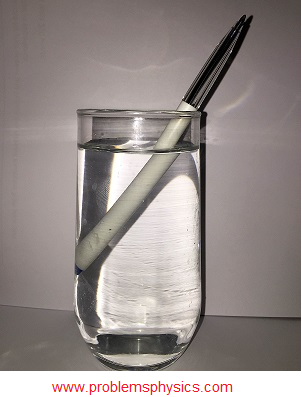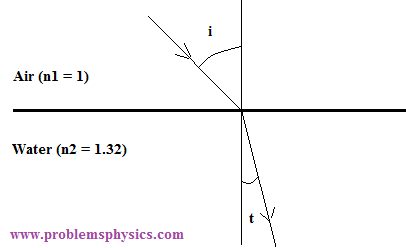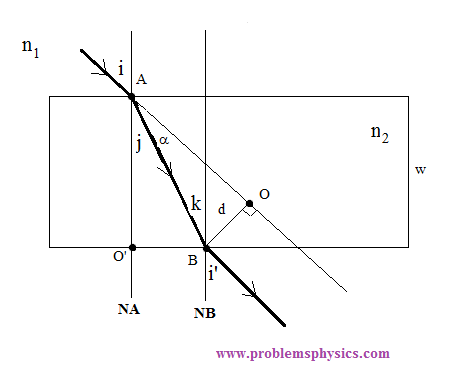# Refraction of Light Rays, Examples and Solutions

#### Refraction of Light Rays at Interfaces

Light rays travel in different mediums at different speeds. In vacuum, for example, light travels at the speed of 3�108 m/s. This is the highest speed possible in physics.

One of the most important parameters that measures optical properties of a medium is the index of refraction. It is defined as the ratio of the speed of light in vacuum (3�108 m/s) and the speed of light v in the medium in question.
The refractive index measure the optical density of a given material. A material with higher refractive is more optically dense than a material with lower refractive index.

 n = c v

Example 1
What is the index of refraction in a medium where the speed of light is 1.5�108 m/s?

Solution

 n = c v
 = 3�108 m/s 1.5�108 m/s
= 2

Example 2
What is the speed of light in water whose refractive index is 1.33?

Solution
 1.33 = 3�108 m/s v
 v = 3�108 m/s 1.33
≈ 2.26�108 m/s

A homogeneous medium has a constant index of refraction throughout the medium and light travels along straight paths in it. Refraction occurs at the interface or boundary of two media with different refractive indices. It is the bending of light rays when transmitted into the second medium.(see diagram below)Snell's Laws of refraction

1) The incident ray, the refracted ray and the normal to the interface at the point of incidence are all included in the same plane called the plane of incidence.

2) n1 sin (i) = n2 sin (t)

where n1 is the refractive index of medium (1), n2 is the refractive index of medium (2), i is the angle of incidence and t is the angle of refraction as shown in the diagram above.

Refraction explains physical phenomena such the appearance of a pen bent inside a glass of water and the separation of white light into different colors such as the rainbow as shown below.Example 3
A ray of light is incident through glass, with refractive index 1.52, on an interface separating glass and water with refractive index 1.32. What is the angle of refraction if the angle of incidence of the ray in glass is 25 �?Solution Use Snell's law to write: 1.52 sin 25� = 1.32 sin t t = arcsin((1.52/1.32) sin 25) ≈ 29.1 �

Example 4
What should be the angle of incidence of a light ray incident through air on the boundary separating air from water so that the angle of refraction is 30 �?(refractive index of air is 1 and that of water is 1.32)Solution Assume refractive index of air is equal to 1. Use Snell's law to write: 1 sin (i) = 1.32 sin 30� i = arcsin (1.32 sin 30�) ≈ 41.3 �

Example 5
A light ray strikes a homogeneous rectangular block of glass of thickness w at an angle i. The ray emerges at point B at an angle i'. (see diagram below)a) Find i' in terms of i and show that the emerging and incident rays are prallel.
b) Find the lateral displacement which is the distance d between the incident and emerging rays in terms of angle i, the refractive indices n1 and n2 and width w of the block.
c) Use the formula obtained in part b) to calculate d for n1 = 1, n2 = 1.55, w = 3 cm and i = 32�.

 Solution a) Snell's at point A n1 sin i = n2 sin j Snell's law at point B n2 sin k = n1 sin i' The normals NA and NB to the interfaces at A and B are parallel to each other and therefore angles j and k are equal. Hence n1 sin i = n2 sin j = n2 sin k = n1 sin i' Therefor i'= i The incident and emerging have equal angles with the normals therefore the emerging and incident rays are parallel. b) Find d Consider the right triangle ABO. sin α = d / AB Consider triangle ABO' cos j = w / AB or AB = w / cos j α = i - j sin (i - j) = d / AB Substitute AB by w / cos j in the above equation sin(i - j) = d cos j / w d = sin(i - j) W / cos j Use Snell's law n1 sin i = n2 sin j j = arcsin [ (n1/n2) sin i ] Hence d = w sin(i - j) / cos j where j = arcsin [ (n1/n2) sin i ] c) Calculate d for n1 = 1, n2 = 1.55, w = 3 cm and i = 32�. j = arcsin [ (n1/n2) sin i ] = arcsin [ (1/1.55) sin 32�] ≈ 20 � d = 3 sin(32 - 20) / cos 20 ≈ 0.7 cm